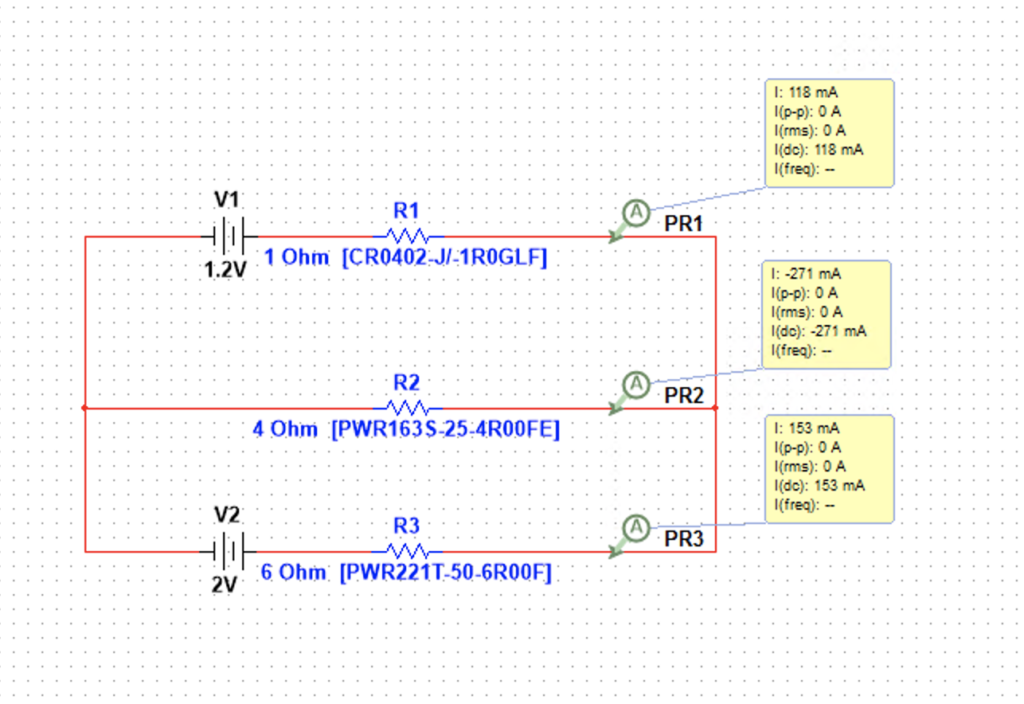# Why Multisim sometime is 100% accurate than calculation, sometimes is not

## Why Multisim sometime is 100% accurate than calculation, sometimes is notThis exercise is 100% same as calculation

But below one is not 100% same as calculation, it use just same components: VC + Resistors. Is multisim using brute force to guess out the voltage and current values?